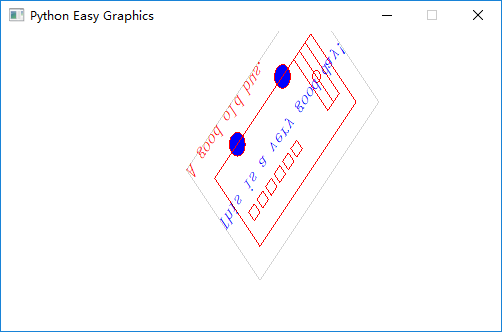# Transforms¶

Easygraphics (and the underlying Qt system) support 5 basic coordinate transform operations:

• Translation: moves every point of a figure or a space by the same distance in a given direction.
• Rotation: the motion of a rigid body around a fixed point
• Scaling :enlarges (increases) or shrinks (diminishes) objects by a separate scale factor for each axis direction.
• Shear Mapping(Skew) : a linear map that displaces each point in fixed direction, by an amount proportional to its signed distance from a line that is parallel to that direction.
• Relection (Flipping): a transformation in geometry in which an object is reflected in a line to form a mirror image.

The following code draws a simple bus. We’ll use it as the basis for this chapter’s examples.

```def draw_bus():
"""
Draw a simple bus
"""
set_color("lightgray")
rect(60, 20, 270, 150)

set_color("red")
set_fill_color("blue")

draw_circle(120, 120, 10)  # draw tyre
draw_circle(200, 120, 10)  # draw tyre
rect(80,40,250,120)

# draw window
x = 90
while x < 175 :
rect(x, 60, x+10, 70)
x += 15

# draw door
rect(220, 60, 240, 120);
line(230, 60, 230, 120);
circle(230, 90, 5);
```

The following program draws a un-transformed bus:

```from easygraphics import *
import draw_bus

def main():
init_graph(500, 300)
draw_bus.draw_bus()
pause()
close_graph()

easy_run(main)
```## Translation¶

Translation is the most commonly used transformation. It moves each point by offset_x on x-axis, and offset_y on y-axis. We use it to move the origin’s position ( and the whole drawing).

The following program use translate() to move the origin to the center of the graphics window, then draw the bus.

```from easygraphics import *
import draw_bus

def main():
init_graph(500, 300)
translate(250, 150)
draw_bus.draw_bus()
pause()
close_graph()

easy_run(main)
```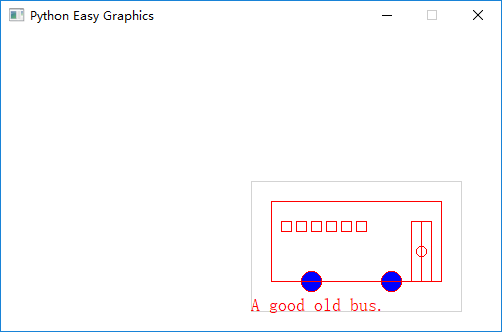Note: set_origin() is an alias of translate()

## Rotation¶

Use rotate() to rotate the coordinate around the point (x,y) clockwise.

Note: the angle directions in rotate() and in shape functions (i.e. draw_pie()) are opposite!

If you need a counter-clockwise rotation, just give a negative rotation degree.

The following program draws a bus rotated 45 degree counter-clockwise around it’s center (105,65).

```from easygraphics import *
import draw_bus

def main():
init_graph(500, 300)
# rotate around the (105,65)
rotate(-45, 105, 65)

draw_bus.draw_bus()
pause()
close_graph()

easy_run(main)
```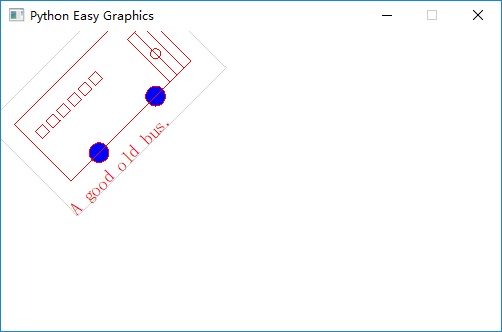## Scaling¶

We can use scale() to scale the drawing in x and y axis separately.

The following program draws a x-axis shrinked and y-axis enlarged bus.

```from easygraphics import *
import draw_bus

def main():
init_graph(500, 300)

scale(0.5, 2)
draw_bus.draw_bus()

pause()
close_graph()

easy_run(main)
```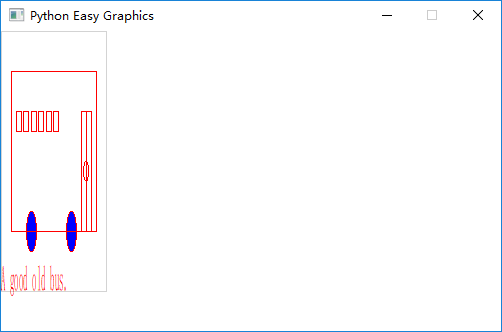## Shear Mapping (Skew)¶

We use shear() or its alias skew to shear a drawing around the center. shear() needs 2 parameters “sv” and “sh”. After shearing, each point (x,y) is transformed to (x+sh*y, y+sv*x). We can see its effect by the following examples.

### Shear on X-axis¶

In the follow example, we shear the bus along the x-axis. Note that the default y-axis is from top to bottom.

```from easygraphics import *
import draw_bus

def main():
init_graph(500, 300)

shear(0.5, 0)
draw_bus.draw_bus()

pause()
close_graph()

easy_run(main)
```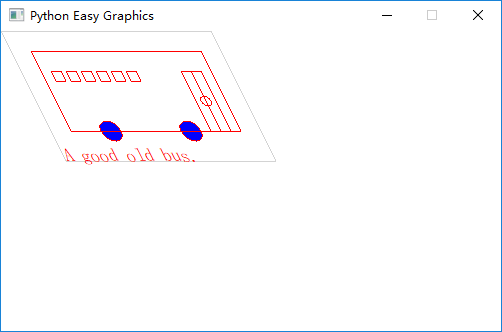### Shear on Y-axis¶

In the follow example, we shear the bus along the y-axis.

```from easygraphics import *
import draw_bus

def main():
init_graph(500, 300)

shear(0, 0.5)
draw_bus.draw_bus()

pause()
close_graph()

easy_run(main)
```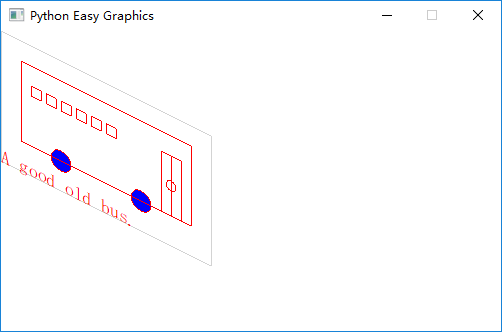### Shear on both axis¶

In the follow example, we shear the bus along the x and y-axis at the same time.

```from easygraphics import *
import draw_bus

def main():
init_graph(500, 300)

shear(0.5, 0.5)
draw_bus.draw_bus()

pause()
close_graph()

easy_run(main)
```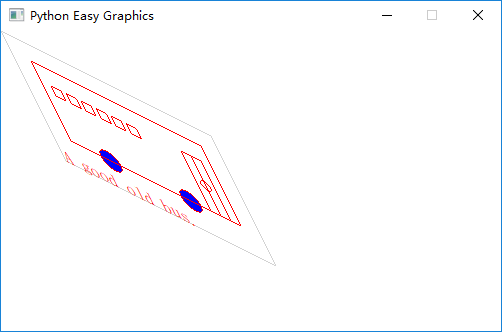## Reflection (Mirror, Flipping)¶

We can use reflect() ( or its alias mirror() and flip() ) to do a reflection. It reflect the drawing again the line passing its parameters (x1,y1) and (x,y).

### Reflection against the y-axis¶

The following program flip the bus horizontally ( reflection against the bus’s vertical center line x=105 ) :

```from easygraphics import *
import draw_bus

def main():
init_graph(500, 300)
reflect(105, 0, 105, 1)
draw_bus.draw_bus()
pause()
close_graph()

easy_run(main)
```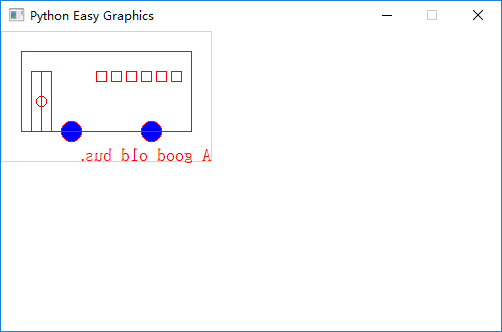### Reflection against the x-axis¶

The following program flip the bus vertically ( reflection against the bus’s horizontal center line y=65 ) :

```from easygraphics import *
import draw_bus

def main():
init_graph(500, 300)

reflect(0, 65, 1, 65)

draw_bus.draw_bus()
pause()
close_graph()

easy_run(main)
```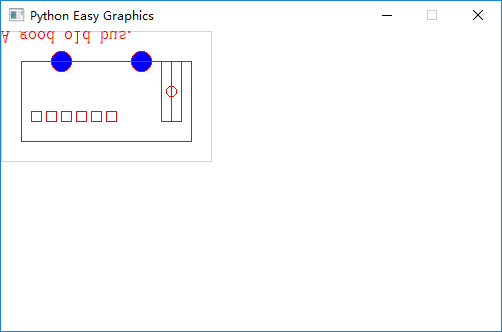### Reflection against other lines¶

The following program flip the bus against the line passing (0, 300) and (500,0). To clearly see the result, we first draw a non-transformed bus, a mirror line, then draw the flipped bus.

```from easygraphics import *
import draw_bus

def main():
init_graph(500, 300)

draw_bus.draw_bus()

set_color("gray")
set_line_style(LineStyle.DASH_LINE)
line(0, 300, 500, 0)
set_line_style(LineStyle.SOLID_LINE)

reflect(0, 300, 500, 0)
draw_bus.draw_bus()
pause()
close_graph()

easy_run(main)
```## Compound Transforms¶

Transforms can be compounded.

In the following example, we first translate the origin to the image center, then rotate the bus around its center, then shear it around its center, then scale it by a factor of 1.2 .

```from easygraphics import *
import draw_bus

def main():
init_graph(500, 300)

# move the origin to the center of the image
translate(250, 150)

# rotate around the bus center
translate(105, 65)
rotate(180)
translate(-105, -65)

# shear arount the bus center
translate(105, 65)
shear(0.5, 0.5)
translate(-105, -65)

# scale
scale(1.2, 1.2)
draw_bus.draw_bus()
pause()
close_graph()

easy_run(main)
```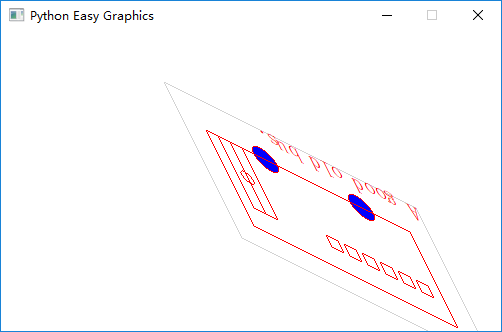## Drawing with Y-Axis Grows Bottom-Up¶

You may have noticed that when you reflect the image, the texts drawing on the image will also get reflected. When what you want is to draw on an ordinary coordinate system whose Y-axis grows bottom-up, this will not be what you what.

Easygraphics provides a set_flip_y() function to used in this situation.

Also notice that if you turn on the set_flip_y(), all the angles parameters used in the drawing functions should be mirrored too. That is, if the docs said a positive angle means turn clock-wise, after the set_flip_y() is on, a positive angle will mean turn counter-clockwise.

Compare the following two programs. The first one use set_flip_y() to make y-axis grows bottom-up; and the second one use reflect(1,0) to do that job. See the results.

Use set_flip_y() to make y-axis grows bottom-up:

```from easygraphics import *
import draw_bus

def main():
init_graph(500, 300)

translate(250, 150)
translate(105, 65)
rotate(-45)
translate(-105, -65)

set_flip_y(True)
# reflect(1,0)

translate(105, -65)
shear(0.2, 0.2)
translate(-105, 65)

draw_bus.draw_bus()
set_color("blue")
draw_rect_text(0, 0, 210, 130, "This is a very good day!")
pause()
close_graph()

easy_run(main)
```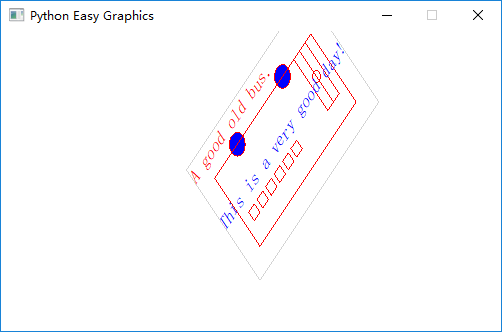Use reflect(1,0) to make y-axis grows bottom-up:

```from easygraphics import *
import draw_bus

def main():
init_graph(500, 300)

translate(250, 150)
translate(105,65)
rotate(-45)
translate(-105,-65)

reflect(1,0)

translate(105, -65)
shear(0.2,0.2)
translate(-105, 65)

draw_bus.draw_bus()
set_color("blue")
draw_rect_text(0,0,210,130,"This is a very good day!")
pause()
close_graph()

easy_run(main)
```